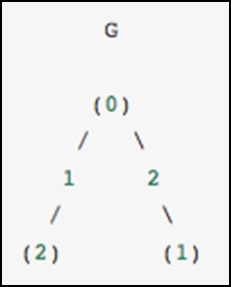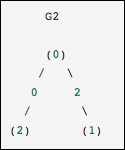# Python - Graph Data

CSGraph stands for Compressed Sparse Graph, which focuses on Fast graph algorithms based on sparse matrix representations.

## Graph Representations

To begin with, let us understand what a sparse graph is and how it helps in graph representations.

### What exactly is a sparse graph?

A graph is just a collection of nodes, which have links between them. Graphs can represent nearly anything − social network connections, where each node is a person and is connected to acquaintances; images, where each node is a pixel and is connected to neighbouring pixels; points in a high-dimensional distribution, where each node is connected to its nearest neighbours and practically anything else you can imagine.

One very efficient way to represent graph data is in a sparse matrix: let us call it G. The matrix G is of size N x N, and G[i, j] gives the value of the connection between node ‘i' and node ‘j’. A sparse graph contains mostly zeros − that is, most nodes have only a few connections. This property turns out to be true in most cases of interest.

The creation of the sparse graph submodule was motivated by several algorithms used in scikit-learn that included the following −

• Isomap − A manifold learning algorithm, which requires finding the shortest paths in a graph.

• Hierarchical clustering − A clustering algorithm based on a minimum spanning tree.

• Spectral Decomposition − A projection algorithm based on sparse graph laplacians.

As a concrete example, imagine that we would like to represent the following undirected graph −This graph has three nodes, where node 0 and 1 are connected by an edge of weight 2, and nodes 0 and 2 are connected by an edge of weight 1. We can construct the dense, masked and sparse representations as shown in the following example, keeping in mind that an undirected graph is represented by a symmetric matrix.

```G_dense = np.array([ [0, 2, 1],
[2, 0, 0],
[1, 0, 0] ])

from scipy.sparse import csr_matrix

G_sparse = csr_matrix(G_dense)
print G_sparse.data
```

The above program will generate the following output.

```array([2, 1, 2, 1])
```This is identical to the previous graph, except nodes 0 and 2 are connected by an edge of zero weight. In this case, the dense representation above leads to ambiguities − how can non-edges be represented, if zero is a meaningful value. In this case, either a masked or a sparse representation must be used to eliminate the ambiguity.

Let us consider the following example.

```from scipy.sparse.csgraph import csgraph_from_dense
G2_data = np.array
([
[np.inf, 2, 0 ],
[2, np.inf, np.inf],
[0, np.inf, np.inf]
])
G2_sparse = csgraph_from_dense(G2_data, null_value=np.inf)
print G2_sparse.data
```

The above program will generate the following output.

```array([ 2., 0., 2., 0.])
```